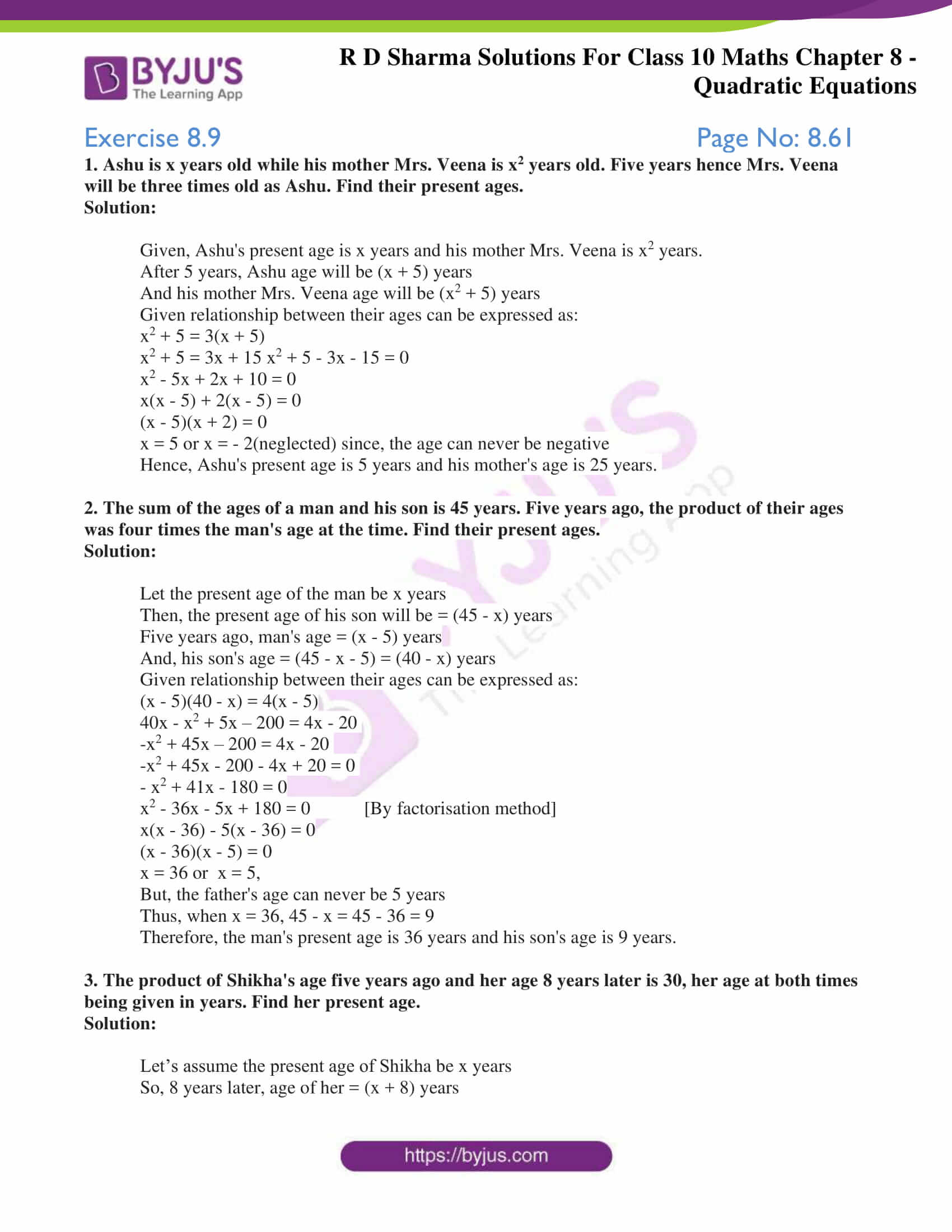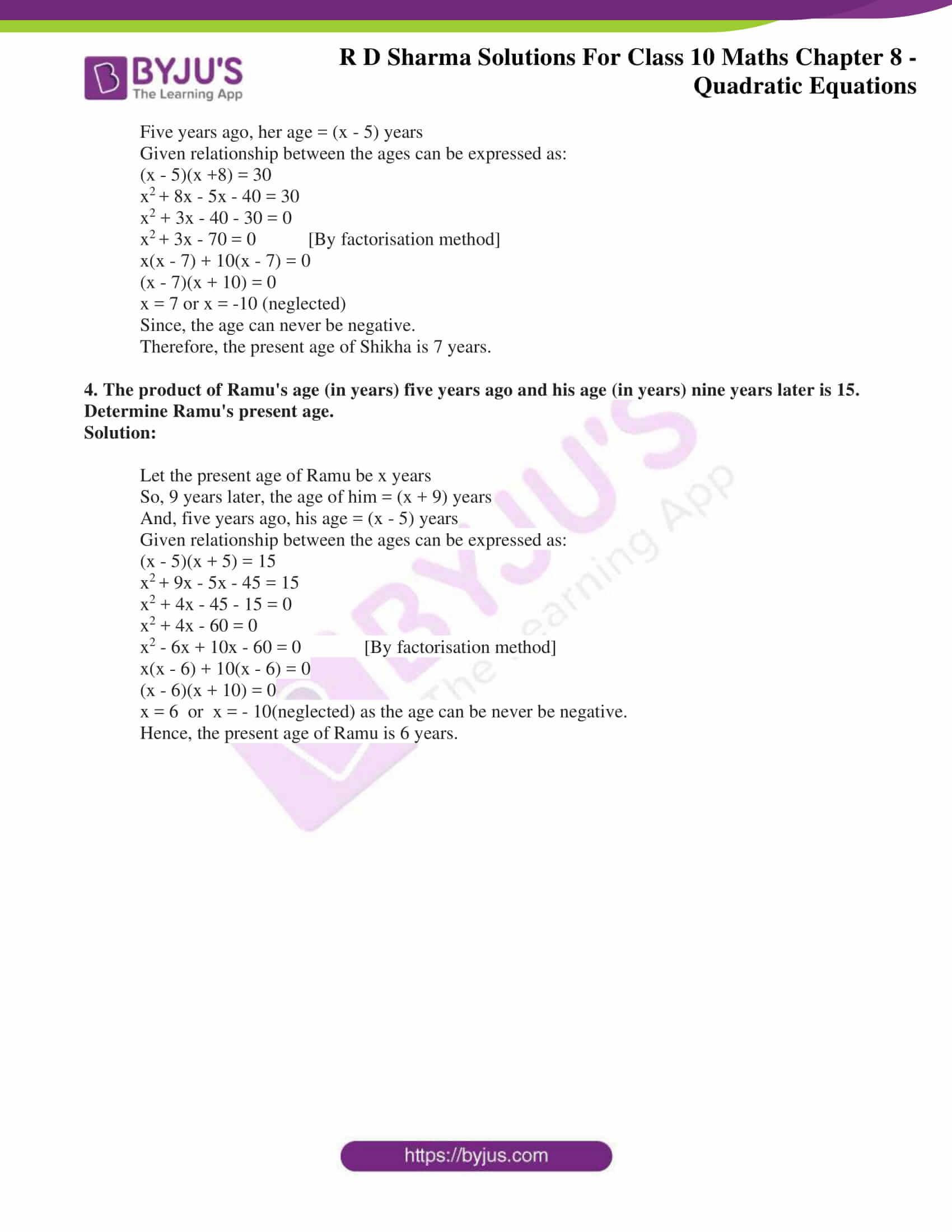# RD Sharma Solutions for Class 10 Maths Chapter 8 Quadratic Equations Exercise 8.9

Another application of quadratic equations is solving problems on ages. Many students get confused in understanding the actual application. That’s why we at BYJU’S have created the RD Sharma Solutions Class 10 containing step by step procedure for solving. Also, the students can access the RD Sharma Solutions for Class 10 Maths Chapter 8 Quadratic Equations Exercise 8.9 PDF given below.### Access RD Sharma Solutions for Class 10 Maths Chapter 8 Quadratic Equations Exercise 8.9

1. Ashu is x years old while his mother Mrs. Veena is x2 years old. Five years hence Mrs. Veena will be three times old as Ashu. Find their present ages.

Solution:

Given, Ashu’s present age is x years and his mother Mrs. Veena is x2 years.

After 5 years, Ashu age will be (x + 5) years

And his mother Mrs. Veena age will be (x2 + 5) years

Given relationship between their ages can be expressed as:

x2 + 5 = 3(x + 5)

x2 + 5 = 3x + 15 x2 + 5 – 3x – 15 = 0

x2 – 5x + 2x + 10 = 0

x(x – 5) + 2(x – 5) = 0

(x – 5)(x + 2) = 0

x = 5 or x = – 2(neglected) since, the age can never be negative

Hence, Ashu’s present age is 5 years and his mother’s age is 25 years.

2. The sum of the ages of a man and his son is 45 years. Five years ago, the product of their ages was four times the man’s age at the time. Find their present ages.

Solution:

Let the present age of the man be x years

Then, the present age of his son will be = (45 – x) years

Five years ago, man’s age = (x – 5) years

And, his son’s age = (45 – x – 5) = (40 – x) years

Given relationship between their ages can be expressed as:

(x – 5)(40 – x) = 4(x – 5)

40x – x2 + 5x – 200 = 4x – 20

-x2 + 45x – 200 = 4x – 20

-x2 + 45x – 200 – 4x + 20 = 0

– x2 + 41x – 180 = 0

x2 – 36x – 5x + 180 = 0 [By factorisation method]

x(x – 36) – 5(x – 36) = 0

(x – 36)(x – 5) = 0

x = 36 or  x = 5,

But, the father’s age can never be 5 years

Thus, when x = 36, 45 – x = 45 – 36 = 9

Therefore, the man’s present age is 36 years and his son’s age is 9 years.

3. The product of Shikha’s age five years ago and her age 8 years later is 30, her age at both times being given in years. Find her present age.

Solution:

Let’s assume the present age of Shikha be x years

So, 8 years later, age of her = (x + 8) years

Five years ago, her age = (x – 5) years

Given relationship between the ages can be expressed as:

(x – 5)(x +8) = 30

x+ 8x – 5x – 40 = 30

x2 + 3x – 40 – 30 = 0

x+ 3x – 70 = 0 [By factorisation method]

x(x – 7) + 10(x – 7) = 0

(x – 7)(x + 10) = 0

x = 7 or x = -10 (neglected)

Since, the age can never be negative.

Therefore, the present age of Shikha is 7 years.

4. The product of Ramu’s age (in years) five years ago and his age (in years) nine years later is 15. Determine Ramu’s present age.

Solution:

Let the present age of Ramu be x years

So, 9 years later, the age of him = (x + 9) years

And, five years ago, his age = (x – 5) years

Given relationship between the ages can be expressed as:

(x – 5)(x + 5) = 15

x+ 9x – 5x – 45 = 15

x2 + 4x – 45 – 15 = 0

x2 + 4x – 60 = 0

x2 – 6x + 10x – 60 = 0 [By factorisation method]

x(x – 6) + 10(x – 6) = 0

(x – 6)(x + 10) = 0

x = 6  or  x = – 10(neglected) as the age can be never be negative.

Hence, the present age of Ramu is 6 years.